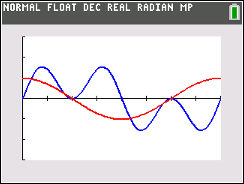### Mathematics lessons for IB® Diploma Programme

Analysis and Approaches | Geometry and Trigonometry

# Can You Solve a Trig Equation?

### Objectives• Students will solve basic multi-step trigonometric equations within a finite interval.
• Students will review and use trigonometric identities to help in the solving of trigonometric equations.
• Students will try to make a connection with how to understand these topics in IB Mathematics courses and on their final assessments.

### Vocabulary

• Finite Interval
• Identity
• Verify